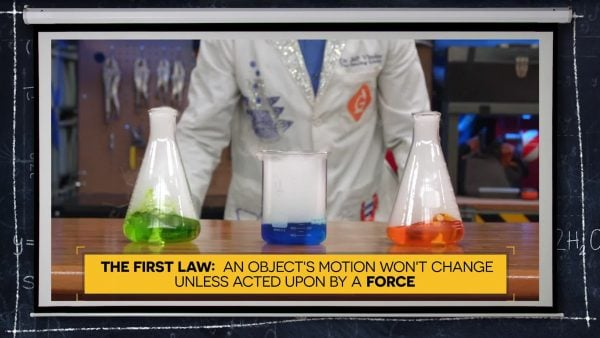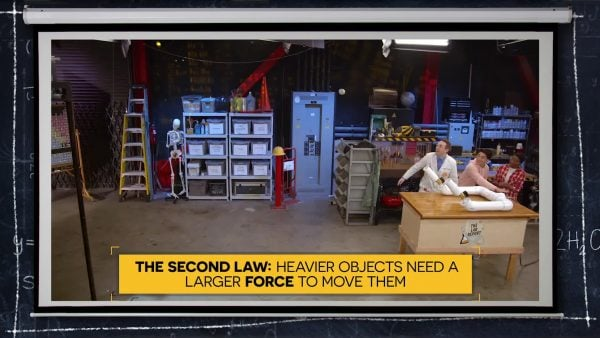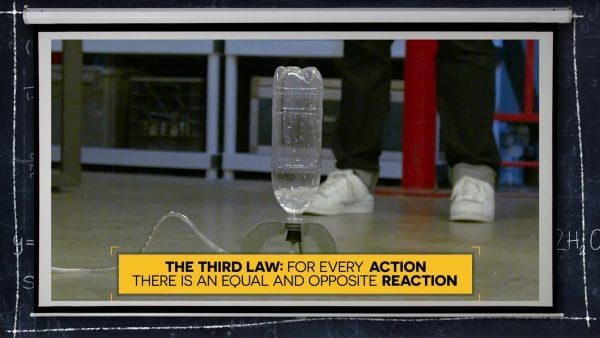Read About Newton's Laws of Motion | Science for Grades 6-8 [Printable]
1%
It was processed successfully!WHAT ARE NEWTON’S LAWS OF MOTION?

There are three laws of motion. These laws were published by Isaac Newton in 1687. Newton’s 1st law tells us that an object won’t change its motion unless acted upon by a force. Newton’s 2nd law tells us that heavier objects need a larger force to move them. Newton’s 3rd law tells us that for every action there is an equal and opposite reaction.

To better understand Newton’s Laws of Motion…

WHAT ARE NEWTON’S LAWS OF MOTION?. There are three laws of motion. These laws were published by Isaac Newton in 1687. Newton’s 1st law tells us that an object won’t change its motion unless acted upon by a force. Newton’s 2nd law tells us that heavier objects need a larger force to move them. Newton’s 3rd law tells us that for every action there is an equal and opposite reaction. To better understand Newton’s Laws of Motion…

## LET’S BREAK IT DOWN!

### Newton’s First Law of MotionNewton’s first law of motion states that objects will continue what they are doing, either staying still or moving, unless they are acted upon by a force. If a ball is sitting still on the grass, it will continue to sit still unless a force, such as a kick from a foot, causes it to move. Likewise, if an object is in motion, such as a rock rolling down a hill, it will continue its motion unless it is interrupted by a force such as a collision with a tree.

Newton’s First Law of Motion Newton’s first law of motion states that objects will continue what they are doing, either staying still or moving, unless they are acted upon by a force. If a ball is sitting still on the grass, it will continue to sit still unless a force, such as a kick from a foot, causes it to move. Likewise, if an object is in motion, such as a rock rolling down a hill, it will continue its motion unless it is interrupted by a force such as a collision with a tree.

### Newton’s Second Law of MotionNewton’s second law of motion states that more force is required to move a heavier object the same distance as a lighter object. Imagine there are two children on a swing set, and one is only 3 and weighs 35 pounds, while the other is 8 and weighs 70 pounds. If their mom pushes them with equal force, the second law of motion says that the heavier child will not go as high as the lighter child. For the heavier child to go as high as the lighter child, their mom would have to push the heavier child with more force.

Newton’s Second Law of Motion Newton’s second law of motion states that more force is required to move a heavier object the same distance as a lighter object. Imagine there are two children on a swing set, and one is only 3 and weighs 35 pounds, while the other is 8 and weighs 70 pounds. If their mom pushes them with equal force, the second law of motion says that the heavier child will not go as high as the lighter child. For the heavier child to go as high as the lighter child, their mom would have to push the heavier child with more force.

### Newton’s Third Law of MotionNewton’s third law of motion states that for every action there is an opposite and equal reaction. This can be explained in many observations. Take a rocket for example. A rocket has a force via a chemical reaction that pushes down on the Earth. This results in the opposite reaction of moving the rocket straight up. Another example is a bouncing ball. As it is pushed down onto the ground, it bounces back up with an equal and opposite reaction.

Newton’s Third Law of Motion Newton’s third law of motion states that for every action there is an opposite and equal reaction. This can be explained in many observations. Take a rocket for example. A rocket has a force via a chemical reaction that pushes down on the Earth. This results in the opposite reaction of moving the rocket straight up. Another example is a bouncing ball. As it is pushed down onto the ground, it bounces back up with an equal and opposite reaction.

### There are several forces we cannot see.Some forces are not as obvious as pushing and pulling objects. Forces such as gravity, friction, and air resistance all impact the motion of objects. One example of this is when a ball is rolling on the ground. It doesn’t roll forever because the force of gravity is pulling it down, and there is friction between the ball and the ground that works to slow it down. Another example is a feather floating to the ground. The force of gravity pulls it down and the force of air resistance pushes up on it and slows it down.

There are several forces we cannot see. Some forces are not as obvious as pushing and pulling objects. Forces such as gravity, friction, and air resistance all impact the motion of objects. One example of this is when a ball is rolling on the ground. It doesn’t roll forever because the force of gravity is pulling it down, and there is friction between the ball and the ground that works to slow it down. Another example is a feather floating to the ground. The force of gravity pulls it down and the force of air resistance pushes up on it and slows it down.

### Many Careers Use Newton’s Laws of MotionSeveral different careers use Newton’s Laws of Motion. One example is engineers. Engineers such as crash test engineers use the laws of motion to help them learn how car collisions affect people. This enables the engineers to develop protective technology such as airbags and place them in the right places in cars. Other engineers such as aerospace engineers use the laws of motion when designing airplanes or space vehicles.

Many Careers Use Newton’s Laws of Motion Several different careers use Newton’s Laws of Motion. One example is engineers. Engineers such as crash test engineers use the laws of motion to help them learn how car collisions affect people. This enables the engineers to develop protective technology such as airbags and place them in the right places in cars. Other engineers such as aerospace engineers use the laws of motion when designing airplanes or space vehicles.

## NEWTON'S LAWS OF MOTION VOCABULARY

Force
A push or pull acting on an object.
Newton’s 1st Law of Motion
This law of motion states that an object’s motion won’t change unless acted upon by a force.
Newton’s 2nd Law of Motion
This law of motion states that heavier objects need a larger force to make them move.
Newton’s 3rd Law of Motion
This law of motion states that for every action there is an opposite and equal reaction.
Gravity
A force that pulls objects down towards the Earth.
Friction
A force resulting from two objects rubbing against each other.
A force caused by air, acting in the opposite direction of a moving object.
The speeding up of an object.
When two forces on an object cancel each other out, they do not affect the motion of the object.
When one force is stronger than other forces on an object, they do affect the motion of an object.

## NEWTON'S LAWS OF MOTION DISCUSSION QUESTIONS

### Newton’s first law of motion states that an object's motion remains the same unless a force acts upon it. What would be an example of this law in action?

If a ball is still, it will remain still until a force, such as a kick, makes it move.

### Newton’s second law of motion states that larger things need more force to move them than lighter things. What would be an example of this law in action?

One example is pushing a car or pushing a shopping cart. You would need much more force to push a car the same distance as a shopping cart.

### Why do people need to know about Newton’s Laws of Motion?

Newton’s laws of motion are helpful when making predictions of motion. This might be important if you were trying to step off a boat onto a dock. If you push off the boat, it will move away from the dock a little, which would be important to know if you don’t want to end up in the water.

### Describe some different forces that act upon objects.

Some more obvious forces might be pushes, kicks or pulls. Some less obvious forces are friction, gravity and air resistance. All these forces affect the movement of objects.

### Newton’s third law of motion states that for every action there is an equal and opposite reaction. What would be an example of this law in action?

An example of this is a ball bouncing. You throw it down on the ground with a force, and it bounces back with an equal force.

### Why don’t objects in motion continue moving forever?

Newton’s 1st law of motion says that an object in motion stays in motion unless a force acts upon it. Even if nothing appears to stop a moving object, forces such as friction, air resistance and gravity are all acting on the object to slow it down.
X

## Success

We’ve sent you an email with instructions how to reset your password.
Ok

## Choose Your Free Trial Period### 7 DaysContinue to Lessons### 90 DaysGet 90 days free by inviting other teachers to try it too.

Share with Teachers

## Get 90 Days Free

### By inviting 4 other teachers to try it too.

4 required

Skip, I will use a 7 days free trial

## Thank You!

Enjoy your free 90 days trial